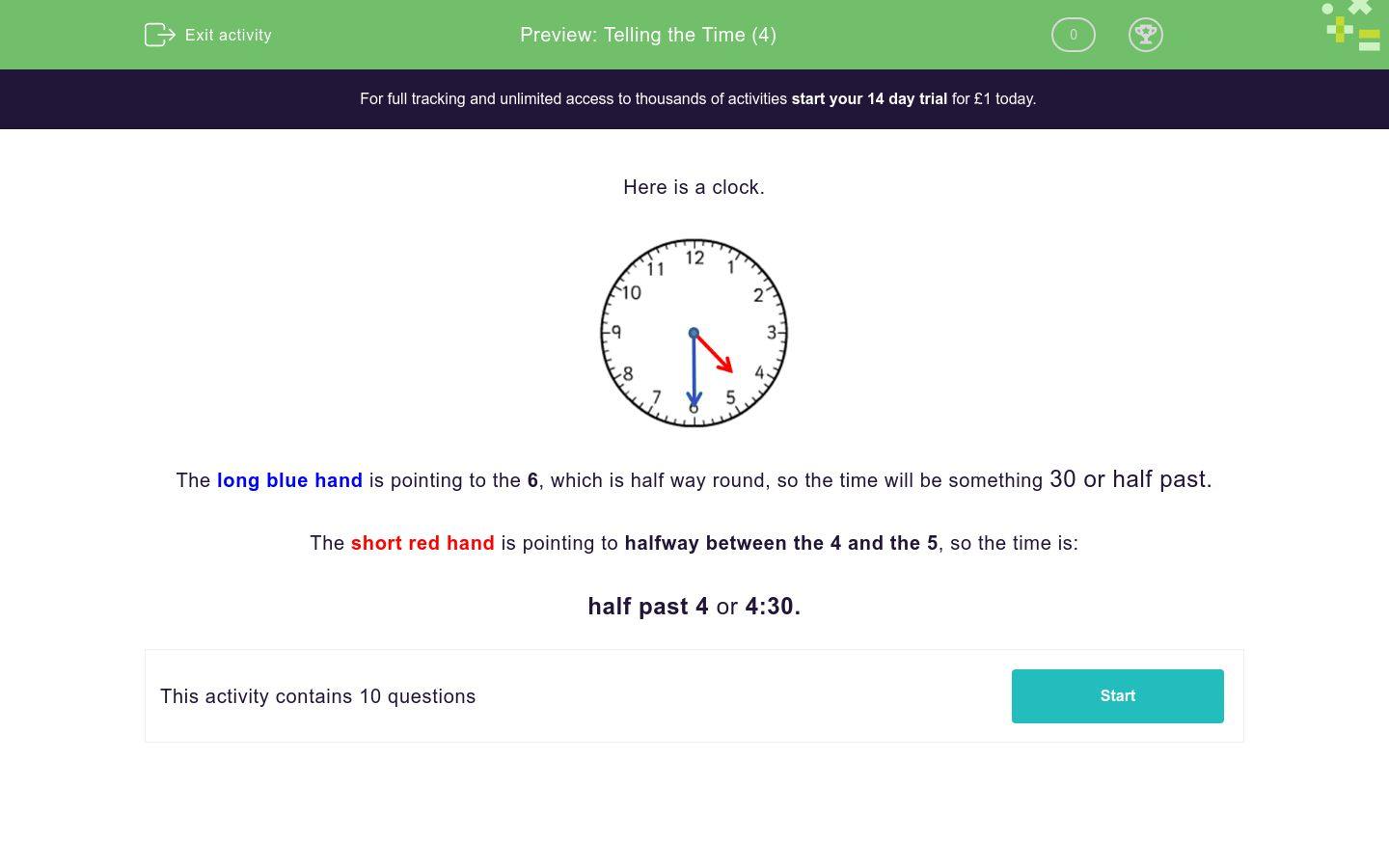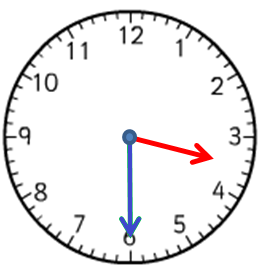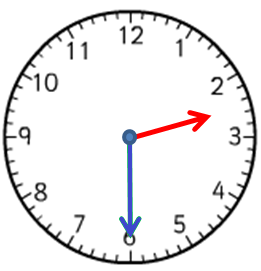# Telling the Time (4)

In this worksheet, students must select the clock face that matches the given time. Only half past times are shown.Key stage:  KS 1

Curriculum topic:   Measurement

Curriculum subtopic:   Tell the Time

Difficulty level:### QUESTION 1 of 10

Here is a clock.The long blue hand is pointing to the 6, which is half way round, so the time will be something 30 or half past.

The short red hand is pointing to halfway between the 4 and the 5, so the time is:

half past 4 or 4:30.

The time is

7:30

Which clock face shows this time?A B C
A

B

C

The time is

2:30

Which clock face shows this time?A B C
A

B

C

The time is

half past 10.

Which clock face shows this time?A B C
A

B

C

The time is

5:30

Which clock face shows this time?A B C
A

B

C

The time is

half past 3.

Which clock face shows this time?A B C
A

B

C

The time is

4:30

Which clock face shows this time?A B C
A

B

C

The time is

1:30

Which clock face shows this time?A B C
A

B

C

The time is

9:30

Which clock face shows this time?A B C
A

B

C

The time is

half past 6.

Which clock face shows this time?A B C
A

B

C

The time is

12:30

Which clock face shows this time?A B C
A

B

C

• Question 1

The time is

7:30

Which clock face shows this time?A B C
A
EDDIE SAYS
The short hand is pointing to halfway between the 7 and the 8.
The long hand is pointing to 6.
• Question 2

The time is

2:30

Which clock face shows this time?A B C
A
EDDIE SAYS
The short hand is pointing to halfway between the 2 and the 3.
The long hand is pointing to 6.
• Question 3

The time is

half past 10.

Which clock face shows this time?A B C
C
EDDIE SAYS
The short hand is pointing to halfway between the 10 and the 11.
The long hand is pointing to 6.
• Question 4

The time is

5:30

Which clock face shows this time?A B C
C
EDDIE SAYS
The short hand is pointing to halfway between the 5 and the 6.
The long hand is pointing to 6.
• Question 5

The time is

half past 3.

Which clock face shows this time?A B C
B
EDDIE SAYS
The short hand is pointing to halfway between the 3 and the 4.
The long hand is pointing to 6.
• Question 6

The time is

4:30

Which clock face shows this time?A B C
B
EDDIE SAYS
The short hand is pointing to halfway between the 4 and the 5.
The long hand is pointing to 6.
• Question 7

The time is

1:30

Which clock face shows this time?A B C
A
EDDIE SAYS
The short hand is pointing to halfway between the 1 and the 2.
The long hand is pointing to 6.
• Question 8

The time is

9:30

Which clock face shows this time?A B C
A
EDDIE SAYS
The short hand is pointing to halfway between the 9 and the 10.
The long hand is pointing to 6.
• Question 9

The time is

half past 6.

Which clock face shows this time?A B C
B
EDDIE SAYS
The short hand is pointing to halfway between the 6 and the 7.
The long hand is pointing to 6.
• Question 10

The time is

12:30

Which clock face shows this time?A B C
A
EDDIE SAYS
The short hand is pointing to halfway between the 12 and the 1.
The long hand is pointing to 6.
---- OR ----

Sign up for a £1 trial so you can track and measure your child's progress on this activity.

### What is EdPlace?

We're your National Curriculum aligned online education content provider helping each child succeed in English, maths and science from year 1 to GCSE. With an EdPlace account you’ll be able to track and measure progress, helping each child achieve their best. We build confidence and attainment by personalising each child’s learning at a level that suits them.

Get started